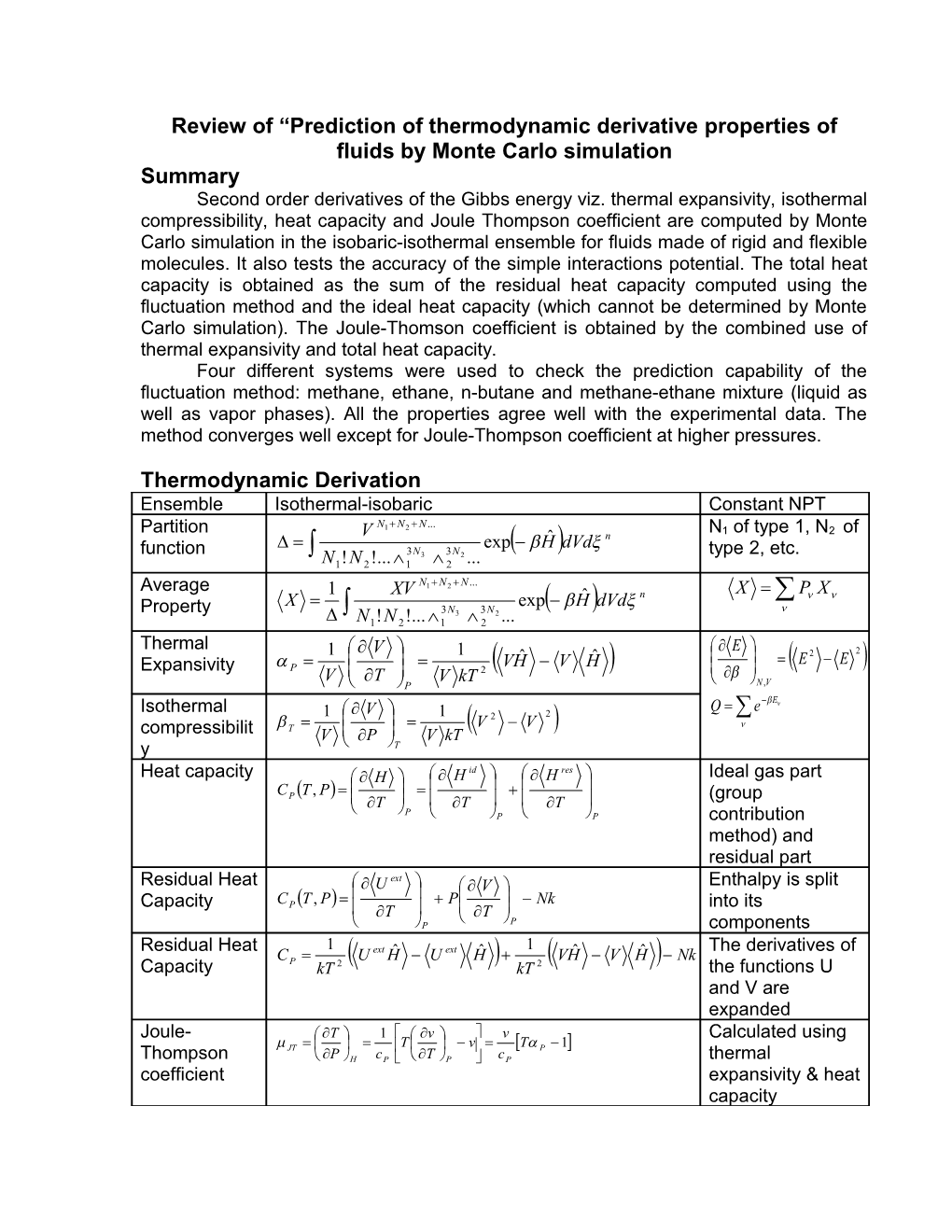# Summary of Prediction of Thermodynamic Derivative Properties of Fluids by Monte Carlo SimulationReview of “Prediction of thermodynamic derivative properties of fluids by Monte Carlosimulation

Summary

Second order derivatives of the Gibbs energy viz. thermal expansivity, isothermal compressibility, heat capacity and Joule Thompson coefficient are computed by Monte Carlo simulation in the isobaric-isothermal ensemble for fluids made of rigid and flexible molecules. It also tests the accuracy of the simple interactions potential. The total heat capacity is obtained as the sum of the residual heat capacity computed using the fluctuation method and the ideal heat capacity (which cannot be determined by Monte Carlo simulation). The Joule-Thomson coefficient is obtained by thecombined use of thermal expansivity and total heat capacity.

Four different systems were used to check the prediction capability of the fluctuation method: methane, ethane, n-butane and methane-ethane mixture (liquid as well as vapor phases). All the properties agree well with the experimental data. The method converges well except for Joule-Thompson coefficient at higher pressures.

Thermodynamic Derivation

Ensemble / Isothermal-isobaric / Constant NPT
Partition function / / N1 of type 1, N2 of type 2, etc.
Average Property / /
Thermal Expansivity / /
Isothermal compressibility /
Heat capacity / / Ideal gas part (group contribution method) and residual part
Residual Heat Capacity / / Enthalpy is split into its components
Residual Heat Capacity / / The derivatives of the functions U and V are expanded
Joule-Thompson coefficient / / Calculated using thermalexpansivity & heat capacity

Simulation Method:

Monte Carlo Algorithm (Introduction)

This method is based on a sampling of the phase space by generation of random configurations. The simulation starts with an initial configuration. A random change leads to a new configuration which is accepted or refused following a thermodynamic criterion. This acceptance criterion assures that, in the limit of an infinite number of generated configurations, a certain configuration j appears with a probability proportional to its Boltzmann factor exp (−Uj/kBT) with Uj standing for the energy of the configuration j. A new configuration is accepted, if its energy Un is smaller than or equal to that of the old configuration, Uo, Otherwise, the new configuration is rejected and the old one is taken once again.

The system is usually represented by a cubic simulation box in which the molecules are placed. Periodic boundary conditions are applied on the faces of the cube in order to avoid surface effects. This means that each molecule that leaves the box from one side automatically re-enters the box on the opposite. Depending on the properties to be determined and on the parameters fixed in the simulation, different applications of the Monte Carlo method are defined. The NPT ensemble is characterized by one simulation box with the number of molecules N, pressure P and temperature T fixed; the volume V of the box is variable.

Molecular Model

Simulations of saturated hydrocarbons are often based on the united atom model (U.A.). In this model, the hydrogen atoms are not represented explicitly but combined with the carbon atom to which they are bound. This leads to a pseudo-atom or more generally to a centre of force describing CH4, CH3, CH2 or CH. Thus, in the U.A. model methane becomes one single interaction site while higher alkanes are represented by their (carbon) backbone. The energy is then given by bond bending energy Ubend, torsional energy Utors, and Lennard–Jones energy ULJ.

U=Ubend + Utors + ULJ

Methane is represented by the U.A. potential. For ethane and n-butane anisotropic united atoms (A.U.A.) potential is used. The difference is that the Lennard-Jones force centre is shifted from the carbon nucleus.

Conclusion

The use of classical thermodynamics, statistical thermodynamics and Monte Carlo simulation with isothermal-isobaric ensemble enables good prediction of second order derivatives of Gibbs energy.